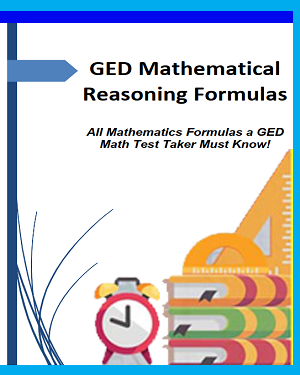# SHOP

GED Mathematical Reasoning Formulas14% Off

### GED Mathematical Reasoning Formulas

#### All Mathematics Formulas a GED Math Test Taker Must Know!

\$6.99 \$5.99
by: Reza Nazari
All Available Formats & Editions
eBook (PDF Format)

Taking the GED with only a few weeks or even few days to study?
First and foremost, you should understand that the 2019 GED® Mathematical Reasoning test contains a formula sheet, which displays formulas relating to geometric measurement and certain algebra concepts. Formulas are provided to test-takers so that they may focus on application, rather than the memorization, of formulas.
However, the test does not provide a list of all basic formulas that will be required to know for the test. This means that you will need to be able to recall many math formulas on the GED.

### The Most Comprehensive GED Math Preparation Bundle

\$79.99 \$34.99
56% Off*

Includes GED Math Prep Books, Workbooks, and Practice Tests

Below you will find the 2019 GED® Mathematics Formula Sheet followed by a complete list of all Math formulas you MUST have learned before test day, as well as some explanations for how to use them and what they mean. Keep this list around for a quick reminder when you forget one of the formulas.

Review them all, then take a look at the math topics to begin applying them!

Good luck!

## Customers Who Bought This Item Also Bought

Total price: \$5.99

## Discover More Book You May Like